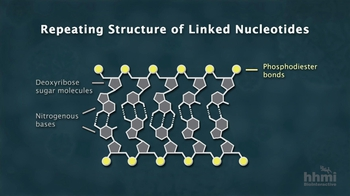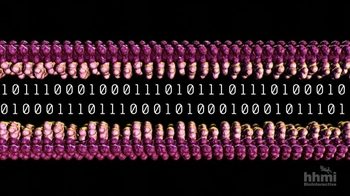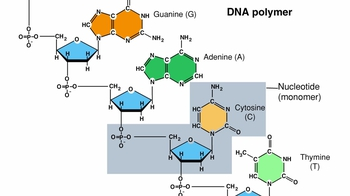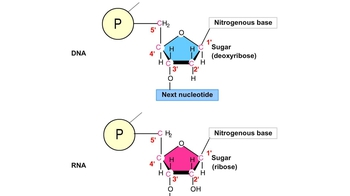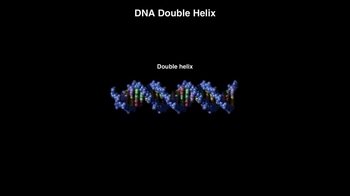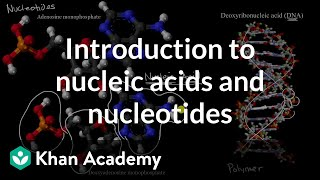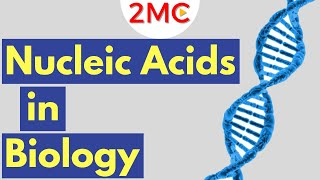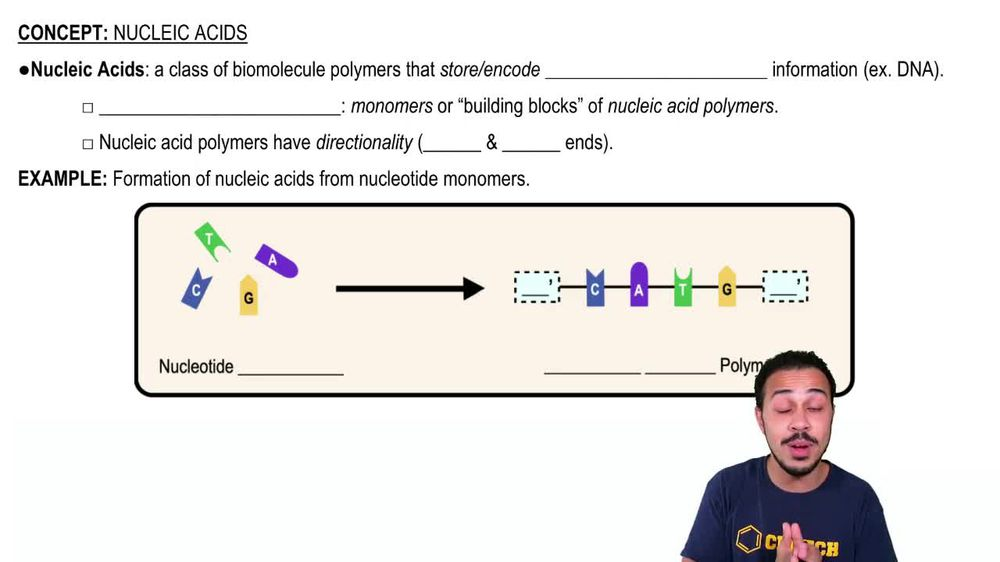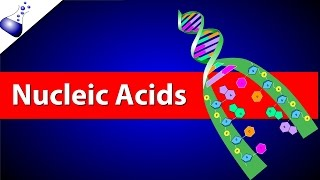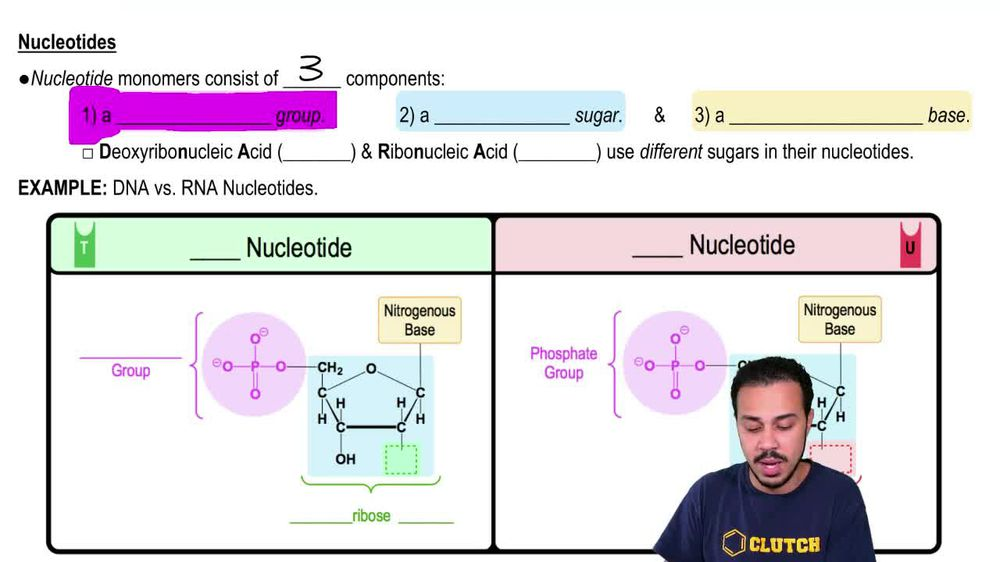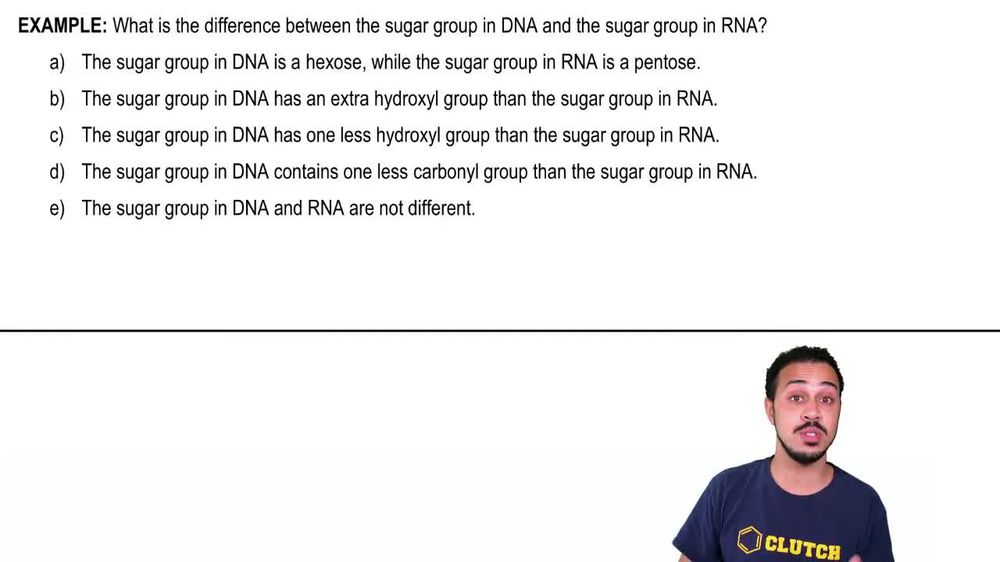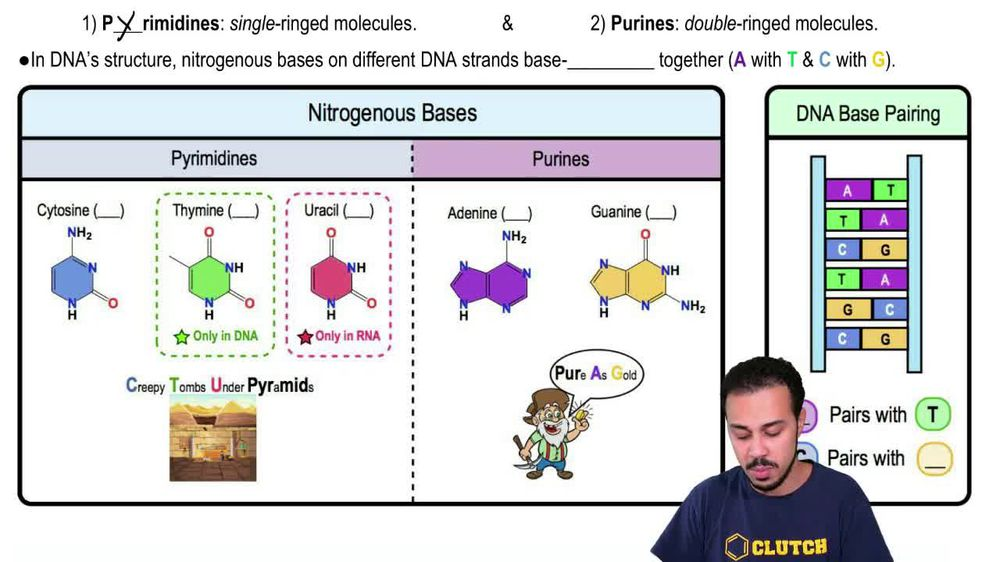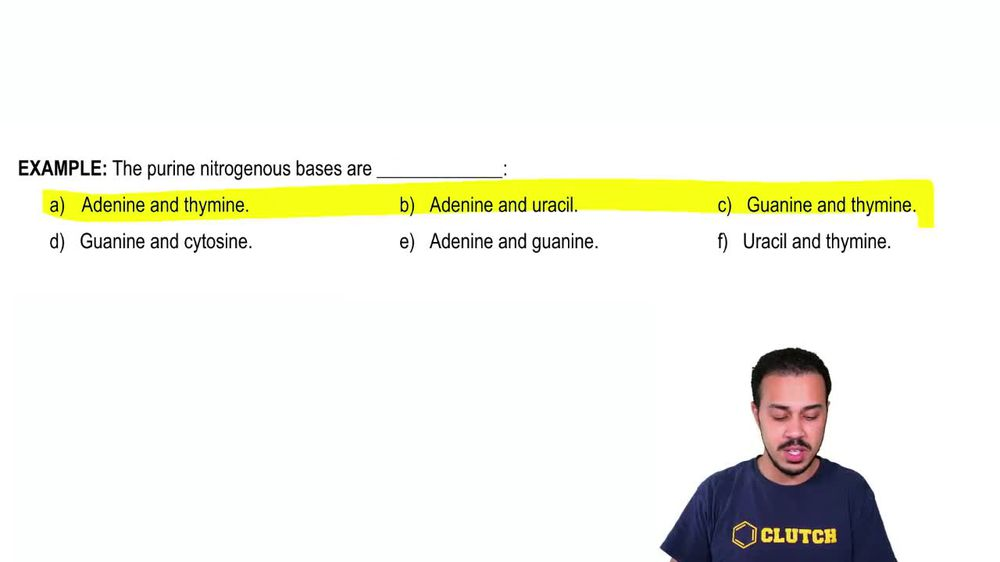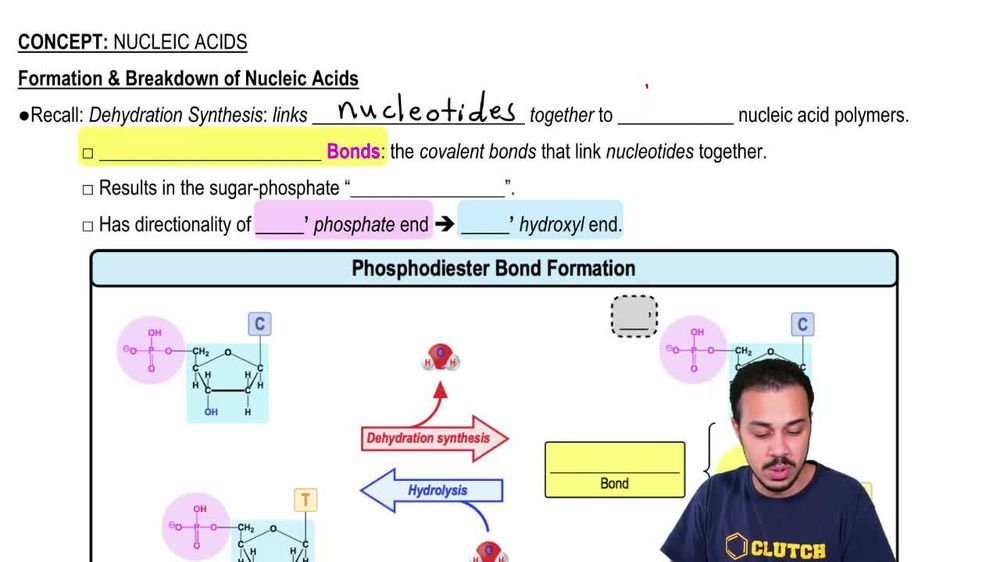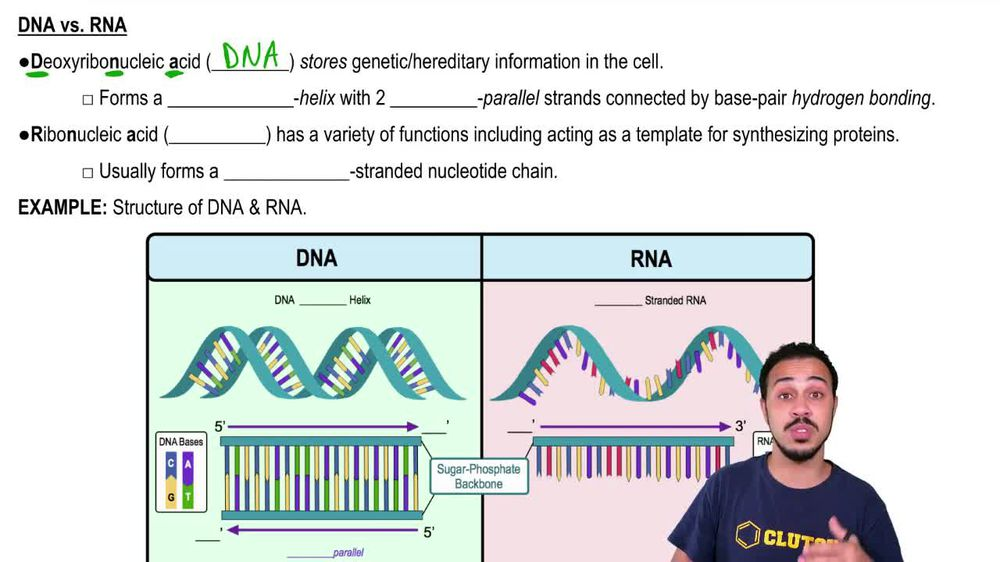Start typing, then use the up and down arrows to select an option from the list.
1. 4. Biomolecules2. Nucleic Acids# Nucleotides

by Jason Amores Sumpter
370 views
6
6
in this video, we're going to talk a little bit more about nucleotides. And so we already know from our last lesson video that nucleotides are the monomers of new click acids. But really, just one single nucleotide monomer consists of three different components that we have number down below here in our text and also noticed that we have the three components color coordinated with these backgrounds, where the first component as a pink background, the second component as a blue background and the third component has a yellow background, and these background colors correspond with the colors that you see down below in our image. And so the very first component of a nucleotide monomer is a phosphate group which, recall from our previous lesson videos, is just a functional group that looks like this down below with a phosphorus atom in the middle. Now, the second component of a nucleotide monomer is a pen, tose, sugar and all. The pintos part, it means, is that this sugar has a five member ring, and so when we take a look at the blue component down below, noticed that there is a ring and the ring has a total of five, uh, members in it, and so that makes us a pento sugar. And then the third and final component of a nucleotide monomer is a nitrogenous base. And so it turns out that the nitrogenous space can vary and there are five different types of nitrogenous bases. And we'll talk more about those five different types of nitrogenous bases and our next lesson video. But for now, taking a look down below, we can see the third component is here in yellow, the nitrogenous base. So in our last lesson video, we were abbreviating nucleotides, using symbols that looks somewhat like this in the corner. But really just one of these nucleotide monomers that we see here in the corner consists of three components. Once again the phosphate group here, the sugar component here and the nitrogenous base up here. And so you can think that this little nucleotide consists of these three components, like what you see here Now this leads us to different types of nucleotides. There are d n a nucleotides or D oxy ribonucleic acid nucleotides. So DNA is the abbreviation for the molecule called D oxy ribonucleic acid on. Then there are also ribonucleic acid nucleotides or R n a nucleotides. And so the DNA and the RNA nucleotides they use different sugars amongst also sometimes using different nitrogenous bases, which will talk more about as we move forward in our course. But if we take a look at our image down below, notice that we're comparing DNA nucleotides versus are in a nucleotides. So the left half of our image over here is specifically for the d n a nucleotide and the right half of our image over here specifically for the r n A nucleotides. So notice that both nucleotides have three components that we talked about before the pink component here, which is the phosphate group. Uh, this one also has a phosphate group, the RNA nuclear type. They both also have a pen tose sugar on dso. You can see that the blue part is here, and then they both also have nitrogenous bases. Now, here's specifically we've said that the nucleotides of DNA and RNA use different sugar. So we're gonna focus in on the blue part here and over here and notice that, uh, the RNA over here on the right, uh, it uses a ribose sugar whereas the DNA over here on the left uses a d oxy ribose sugar. And so the D oxy part here means one less oxygen. Oxy means oxygen and d means without. So the deoxyribonucleic sugar has one less oxygen in comparison to the ribose sugar over here. And so what this means is that the ribose sugar is gonna have a hydroxyl group in O H group at this position, and the deoxyribonucleic over here is not gonna have the extra oxygen is just gonna have a hydrogen here. And so the d Oxy Ribas has one less oxygen. Comparing this position to this position over here. And so we'll get to talk Maura about DNA and RNA as we move forward in our course. But again, one of the biggest difference is is that they use different sugars. Uh, DNA uses deoxyribonucleic sugar, and Arna uses ribose sugar. So this year concludes our introduction to nucleotides, and we'll be able to get some practice applying these concepts as we move forward in our course. So I'll see you in our next video